# Matching Supply with Demand

## Quiz 3 :Estimating and Reducing Labor CostsLooking for Management Homework Help?

## Quiz 3 :Estimating and Reducing Labor Costs(Empty System, Labor Utilization) Consider a process consisting of three resources in a worker-paced line and a wage rate of $10 per hour. Assume there is unlimited demand for the product.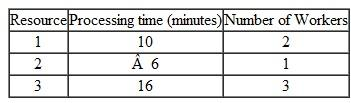a. How long does it take the process to produce 100 units starting with an empty system b. What is the average labor content c. What is the average labor utilization d. What is the cost of direct labor Free Essay Answer: Answer: The throughput rate of a set of resources will depend on the capacity of the bottleneck resource. A bottleneck is the resource having the minimum capacity. The capacity of a resource is computed by the following expression: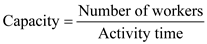a. Compute the capacity of each of the resources by using the following Excel computations.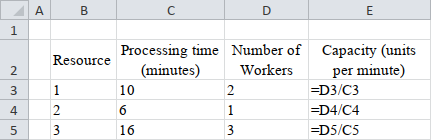Results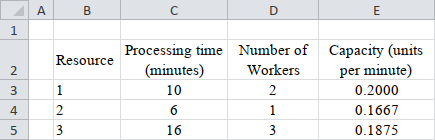Note that the resource 2, having the lowest capacity, is the bottleneck resource. The flow rate will be equal to the capacity of the bottleneck resource i.e. 0.1667 units per minute. Compute the total time to produce 100 units starting with an empty system as follows:So, the total time to complete 100 units is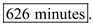b. Compute the average labour content by summing up the processing times at the three resources. So,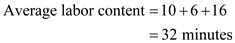The average labour content is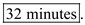c. Compute the average labour utilization using the following formula: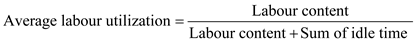Compute the idle time of the workers by subtracting the processing time from the product of the system cycle time and the number of workers. So, The idle time of the workers at resource-1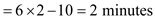The idle time of the workers at resource-2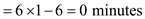The idle time of the workers at resource-3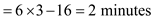So, the total idle time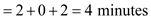Therefore, the average labour utilization is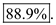d. Compute the cost of direct labour using the following formula given the wage rate being$10 per worker-hour.The cost of direct labour is, therefore,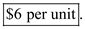(Assign Tasks to Workers) Consider the following six tasks that must be assigned to four workers on a conveyor-paced assembly line (i.e., a machine-paced line flow). Each worker must perform at least one task.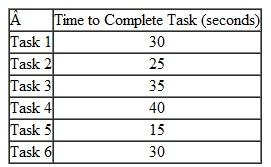The current conveyor-paced assembly line configuration assigns the workers in the following way: • Worker 1: Task 1 • Worker 2: Task 2 • Worker 3: Tasks 3, 4 • Worker 4: Tasks 5, 6 a. What is the capacity of the current line b. Now assume that tasks are allocated to maximize capacity of the line, subject to the conditions that (1) a worker can only perform two adjacent operations and (2) all tasks need to be done in their numerical order. What is the capacity of this line now c. Now assume that tasks are allocated to maximize capacity of the line and that tasks can be performed in any order. What is the maximum capacity that can be achieved
Free
Essay

The throughput rate of a set of resources will depend on the capacity of the bottleneck resource. A bottleneck is the resource having the minimum capacity. The capacity of a resource is computed by the following expression:a.
Compute the capacity of each of the resources by using the following Excel setup and computations.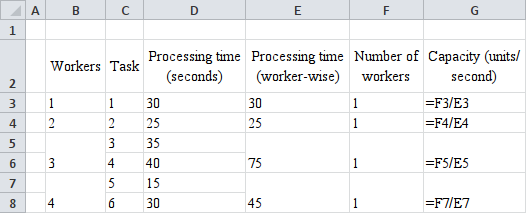Results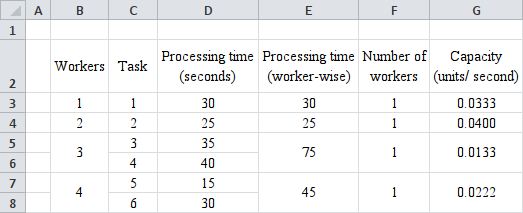Note that the worker-3, having the lowest capacity, is the bottleneck resource. The capacity of the line will be equal to the capacity of the bottleneck resource i.e. 0.0133 units per second.
So, the capacity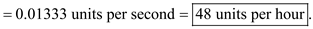b.
Use the following assignments of tasks in order to get the maximum possible capacity given the two constraints.
Compute the capacity of each of the resources by using the following Excel setup and computations.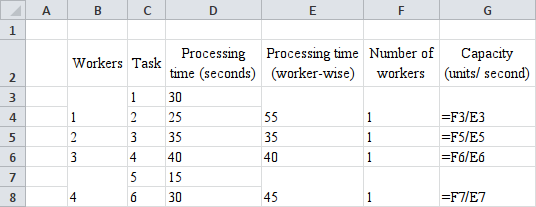Results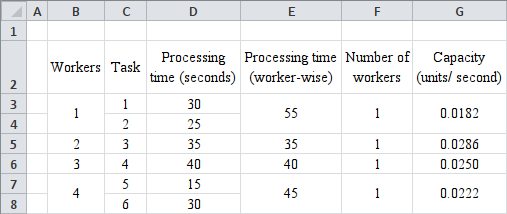Note that the worker-1, having the lowest capacity, is the bottleneck resource. The capacity of the line will be equal to the capacity of the bottleneck resource i.e. 0.0182 units per second.
So, the capacity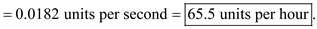c.
Note that there are 4 workers and 6 tasks. So, at least two combinations of tasks must be carried out to accommodate all the tasks. And none of the combinations will give rise to worker-wise processing time less than 55 minutes for the bottleneck. So, it is practically impossible to increase the capacity more than that found in part-b.
So, the capacity even after rearrangement is(PowerToys) PowerToys Inc. produces a small remote-controlled toy truck on a conveyor belt with nine stations. Each station has, under the current process layout, one worker assigned to it. Stations and processing times are summarized in the following table: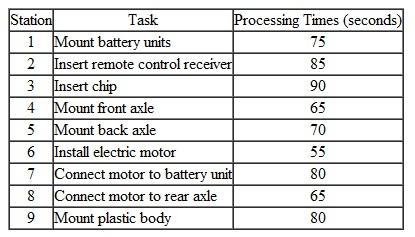a. What is the bottleneck in this process b. What is the capacity, in toy trucks per hour, of the assembly line c. What is the direct labor cost for the toy truck with the current process if each worker receives $15/hour, expressed in dollars per toy truck d. What would be the direct labor cost for the toy truck if work would be organized in a work cell, that is, one worker performs all tasks Assume that the processing times would remain unchanged (i.e., there are no specialization gains). e. What is the utilization of the worker in statioN2 Because of a drastically reduced forecast, the plant management has decided to cut staffing from nine to six workers per shift. Assume that (i) the nine tasks in the preceding table cannot be divided; (ii) the nine tasks are assigned to the six workers in the most efficient way possible; and (iii) if one worker is in charge of two tasks, the tasks have to be adjacent (i.e., one worker cannot work on tasks 1 and 3). f. How would you assign the nine tasks to the six workers g. What is the new capacity of the line (in toy trucks per hour) Free Essay Answer: Answer: The throughput rate of a set of resources will depend on the capacity of the bottleneck resource. A bottleneck is the resource having the minimum capacity. The capacity of a resource is computed by the following expression:a. Compute the capacity of each of the resources by using the following Excel setup and computations.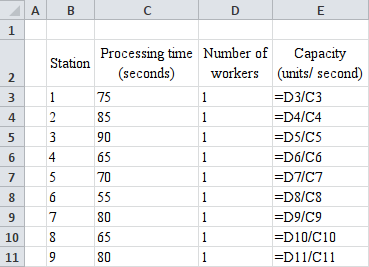Results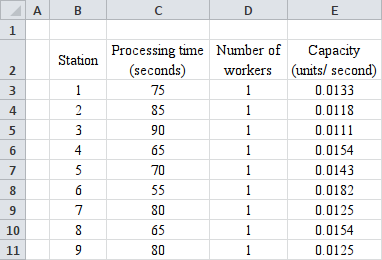Note that the worker working at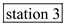, having the lowest capacity, is the bottleneck resource. b. The capacity of the line will be equal to the capacity of the bottleneck resource i.e. 0.011 units per second. So,The capacity is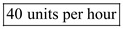. c. Given an unconstrained demand rate, the flow rate will be same as the capacity of the line i.e. 40 per hour. Compute the cost of direct labour using the following formula given the wage rate being$15 per worker-hour.
The cost of direct labour is, therefore,The cost of direct labour is, therefore,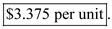d.
If a single worker performs all the tasks, then one unit will be produced in a time which is equal to the total of the processing time of all the 9 stations.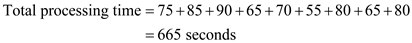So, flow rate will be 1 unit in 665 seconds i.e.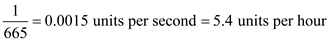The cost of direct labour is, therefore,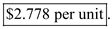e.
Compute the utilization of a resource using the following formula: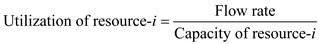So,So, the utilization of workeR2 is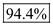f.
There are 6 workers and 9 stations. So, at least 3 pairs of workstations must be combined. The combination pairs are as follows: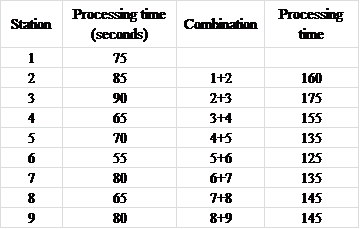The pairs which give the least processing times in ascending order are 5+6, 7+8, and 3+4.
So, the optimal assignments will be as follows: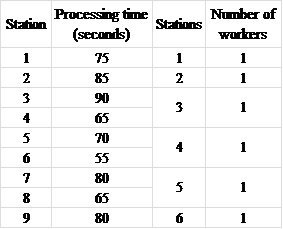g.
Compute the capacity by using the following Excel setup and computations.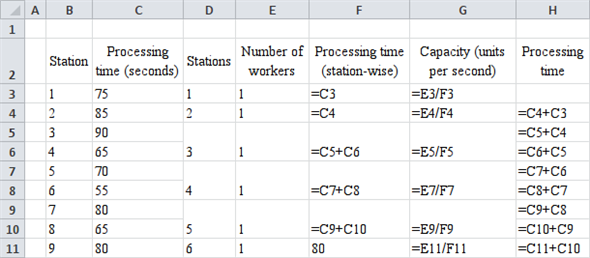Results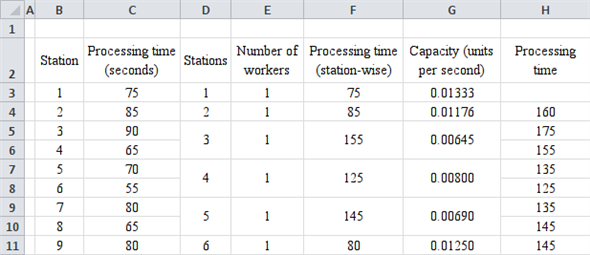Note that the worker working at station 3, having the lowest capacity, is the bottleneck resource.
The capacity is 0.00645 units per second or,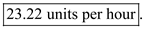(12 Tasks to 4 Workers) Consider the following tasks that must be assigned to four workers on a conveyor-paced assembly line (i.e., a machine-paced line flow). Each worker must perform at least one task. There is unlimited demand.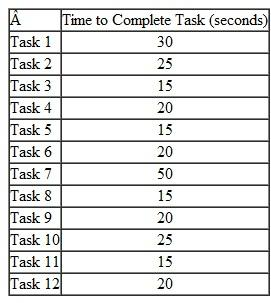The current conveyor-paced assembly-line configuration assigns the workers in the following way: • Worker 1: Tasks 1, 2, 3 • Worker 2: Tasks 4, 5, 6 • Worker 3: Tasks 7, 8, 9 • Worker 4: Tasks 10, 11, 12 a. What is the capacity of the current line b. What is the direct labor content c. What is the average labor utilization (do not consider any transient effects such as the line being emptied before breaks or shift changes) d. How long would it take to produce 100 units, starting with an empty system The firm is hiring a fifth worker. Assume that tasks are allocated to the five workers to maximize capacity of the line, subject to the conditions that (i) a worker can only perform adjacent operations and (ii) all tasks need to be done in their numerical order. e. What is the capacity of this line now Again, assume the firm has hired a fifth worker. Assume further that tasks are allocated to maximize capacity of the line and that tasks can be performed in any order. f. What is the maximum capacity that can be achieved g. What is the minimum number of workers that could produce at an hourly rate of 72 units Assume the tasks can be allocated to workers as described in the beginning (i.e., tasks cannot be done in any order).
Essay(Geneva Watch) The Geneva Watch Corporation manufactures watches on a conveyor belt with six stations. One worker stands at each station and performs the following tasks: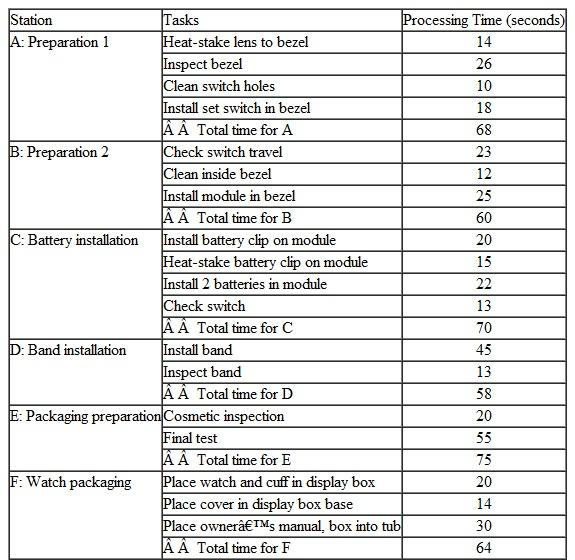These six workers begin their workday at 8:00 a.m. and work steadily until 4:00 p.m. At 4:00, no new watch parts are introduced into station A and the conveyor belt continues until all of the work-in-process inventory has been processed and leaves station F. Thus, each morning the workers begin with an empty system. a. What is the bottleneck in this process b. What is the capacity, in watches per hour, of the assembly line (ignore the time it takes for the first watch to come off the line) c. What is the direct labor content for the processes on this conveyor belt d. What is the utilization of the worker in station B (ignore the time it takes for the first watch to come off the line) e. How many minutes of idle time will the worker in station C have in one hour (ignore the time it takes for the first watch to come off the line) f. What time will it be (within one minute) when the assembly line has processed 193 watches on any given day
Essay(Yoggo Soft Drink) A small, privately owned Asian company is producing a private-label soft drink, Yoggo. A machine-paced line puts the soft drinks into plastic bottles and then packages the bottles into boxes holding 10 bottles each. The machine-paced line is comprised of the following four steps: (1) the bottling machine takes 1 second to fill a bottle, (2) the lid machine takes 3 seconds to cover the bottle with a lid, (3) a labeling machine takes 5 seconds to apply a label to a bottle, and (4) the packaging machine takes 4 seconds to place a bottle into a box. When a box has been filled with 10 bottles, a worker tending the packaging machine removes the filled box and replaces it with an empty box. Assume that the time for the worker to remove a filled box and replace it with an empty box is negligible and hence does not affect the capacity of the line. At step 3 there are two labeling machines that each process alternating bottles, that is, the first machine processes bottles 1, 3, 5,... and the second machine processes bottles 2, 4, 6,... Problem data are summarized in the table following.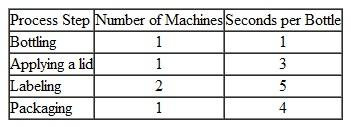a. What is the process capacity (bottles/hour) for the machine-paced line b. What is the bottleneck in the process c. If one more identical labeling machine is added to the process, how much is the increase in the process capacity going to be (in terms of bottles/hour) d. What is the implied utilization of the packaging machine if the demand rate is 60 boxes/ hour Recall that a box consists of 10 bottles.
Essay(Atlas Inc.) Atlas Inc. is a toy bicycle manufacturing company producing a five-inch small version of the bike that Lance Armstrong rode to win his first Tour de France. The assembly line at Atlas Inc. consists of seven work stations, each performing a single step. Stations and processing times are summarized here: • Step 1 (30 sec.): The plastic tube for the frame is cut to size. • SteP2 (20 sec.): The tube is put together. • Step 3 (35 sec.): The frame is glued together. • Step 4 (25 sec.): The frame is cleaned. • Step 5 (30 sec.): Paint is sprayed onto the frame. • Step 6 (45 sec.): Wheels are assembled. • Step 7 (40 sec.): All other parts are assembled to the frame. Under the current process layout, workers are allocated to the stations as shown here: • Worker 1: Steps 1, 2 • Worker 2: Steps 3, 4 • Worker 3: Step 5 • Worker 4: Step 6 • Worker 5: Step 7 a. What is the bottleneck in this process b. What is the capacity of this assembly line, in finished units/hour c. What is the utilization of Worker 4, ignoring the production of the first and last units d. How long does it take to finish production of 100 units, starting with an empty process e. What is the average labor utilization of the workers, ignoring the production of the first and last units f. Assume the workers are paid $15 per hour. What is the cost of direct labor for the bicycle g. Based on recommendations of a consultant, Atlas Inc. decides to reallocate the tasks among the workers to achieve maximum process capacity. Assume that if a worker is in charge of two tasks, then the tasks have to be adjacent to each other. Also, assume that the sequence of steps cannot be changed. What is the maximum possible capacity, in units per hour, that can be achieved by this reallocation h. Again, assume a wage rate of$15 per hour. What would be the cost of direct labor if one single worker would perform all seven steps You can ignore benefits of specialization, set-up times, or quality problems. i. On account of a reduced demand forecast, management has decided to let go of one worker. If work is to be allocated among the four workers such that (i) the tasks can't be divided, (ii) if one worker is in charge of two tasks, the tasks have to be adjacent, (iii) the tasks are assigned in the most efficient way and (iv) each step can only be carried out by one worker, what is the new capacity of the line (in finished units/hour)(Glove Design Challenge) A manufacturer of women's designer gloves has employed a team of students to redesign her manufacturing unit. They gathered the following information. The manufacturing process consists of four activities: (1) fabric cutting; (2) dyeing; (3) stitching, done by specially designed machines; and (4) packaging. Processing times are shown below. Gloves are moved between activities by a conveyor belt that paces the flow of work (machine-paced line).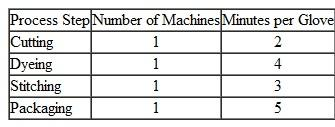a. What is the process capacity in gloves/hour b. Which one of the following statements is true i. The capacity of the process increases by reducing the dyeing time. ii. If stitching time increases to 5 min./glove, the capacity of the process remains unchanged, but "time through an empty machine-paced process" increases. iii. By reducing packaging time, the process capacity increases. iv. By reducing cutting time, the capacity of the process increases. c. What is the implied utilization of the packaging machine if the demand rate is 10 gloves/hour d. What is the flow time for a glove(Worker-Paced Line)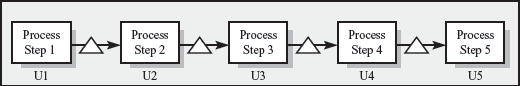The accompanying diagram depicts a five-step, worker-paced headphone manufacturing plant. The headphones are meant to be used with iPods and DVD players. Step 1 involves a worker bending a metal strip into an arc shape. In step 2, the metal arc is fitted with a plastic sleeve. In step 3, the headphones are fitted at the end of the metal and plasticstrips. In step 4, the wires are soldered into the headphones. Step 5 involves a speciallydesigned packaging unit. After the plant has been operational for a couple of hours, themanager inspects the plant. He is particularly interested in cutting labor costs. He observesthe following. The process is capacity constrained and the entire process produces36 units in one hour. U1 through U5 denote the utilization at steps 1 through 5 respectively.Currently, there is a single worker at each step and the utilizations are as follows:U1 = 4/30, U2 = 4/15, U3 = 4/5, U4 = 1, U5 = 2/5. Answer the following questions based on the given data and information. a. What is the capacity of step 5 b. Which step is the bottleneck c. Which process step has the highest capacity d. If the wage rate is \$36 per hour per person, what is the direct labor cost per unit